# PART I.2 - Physical Mathematics

14 de Jan de 2017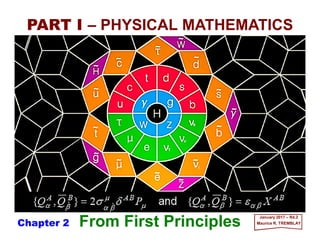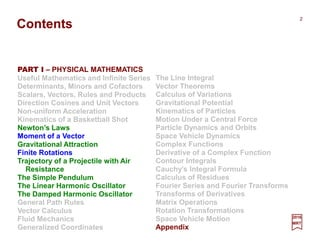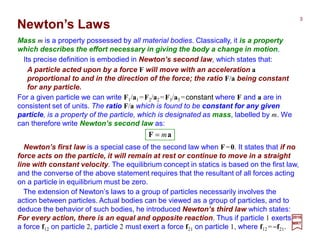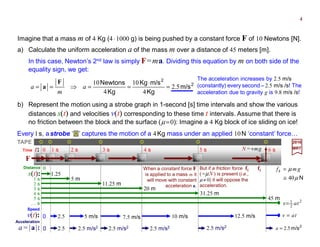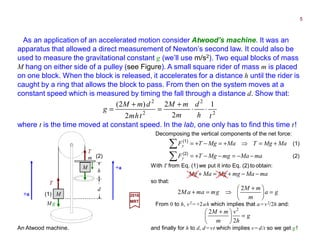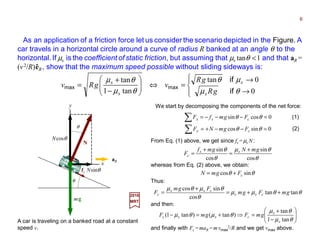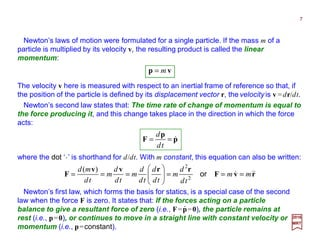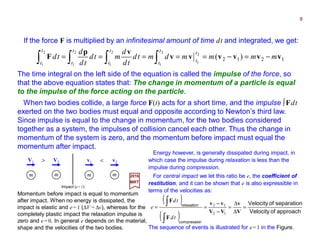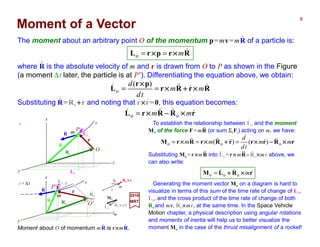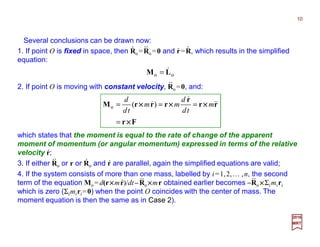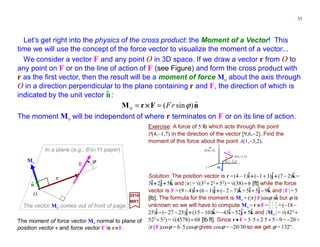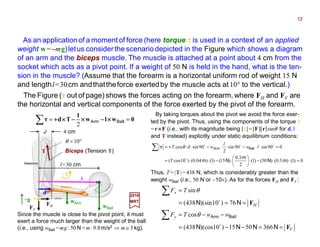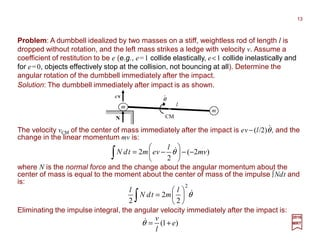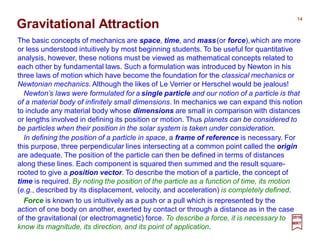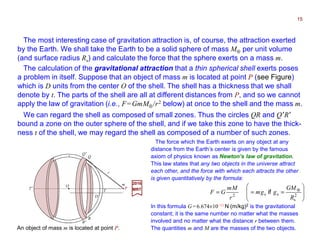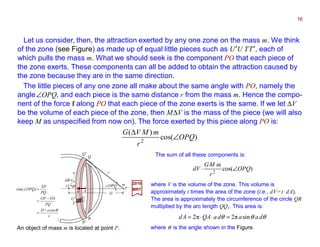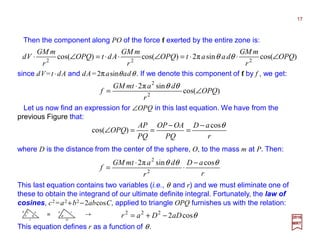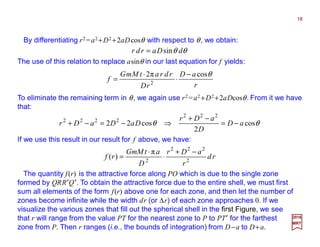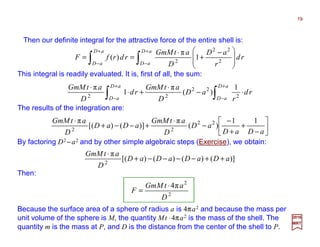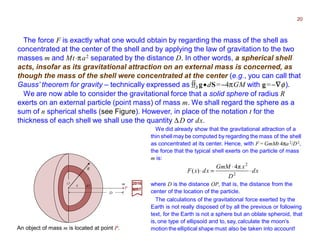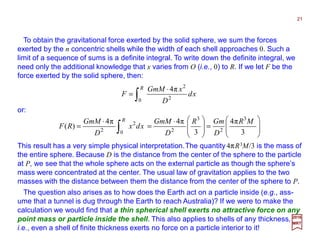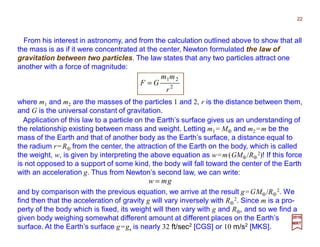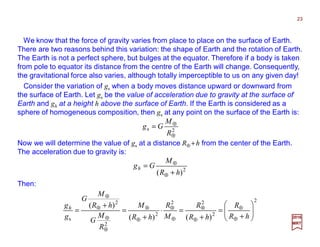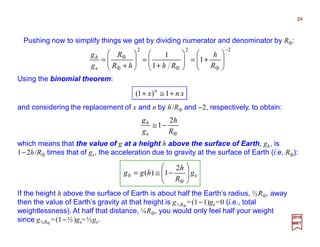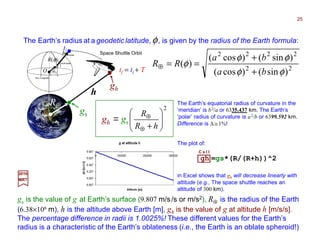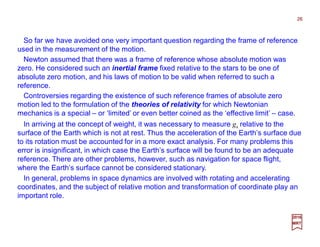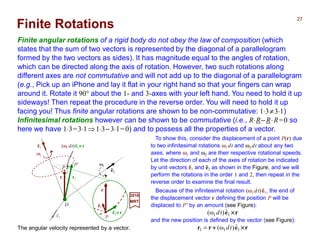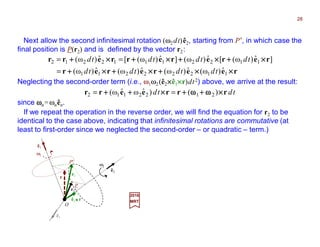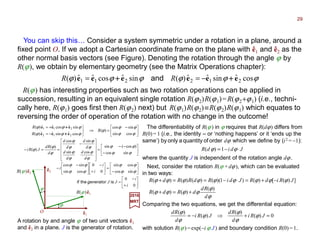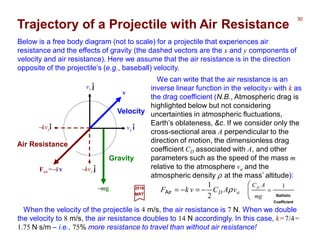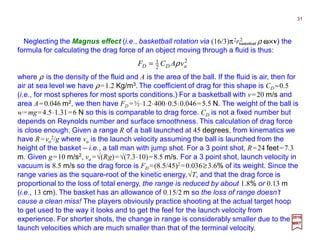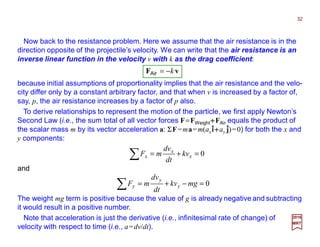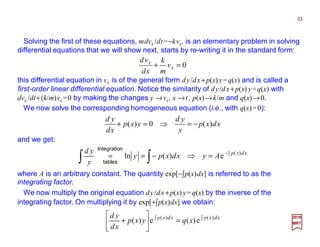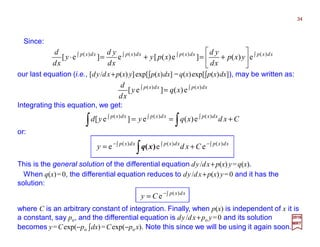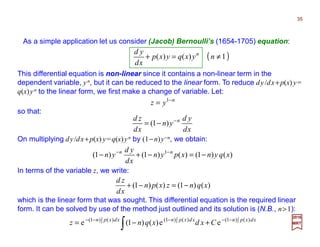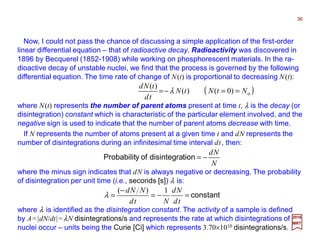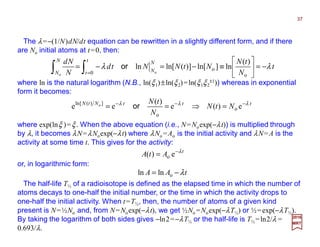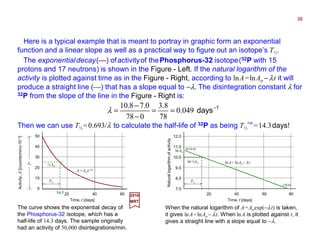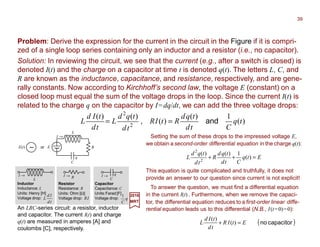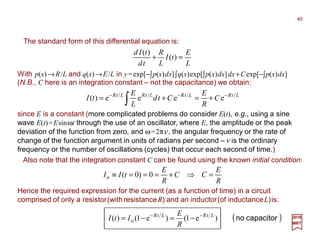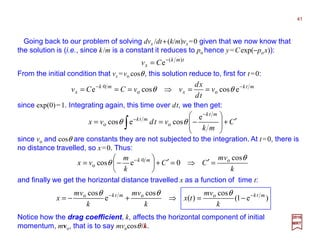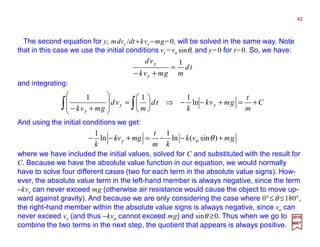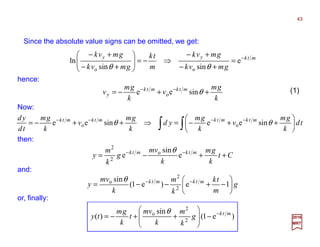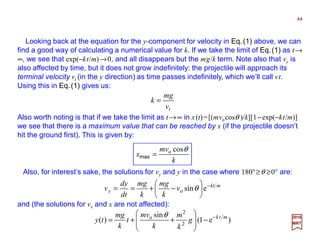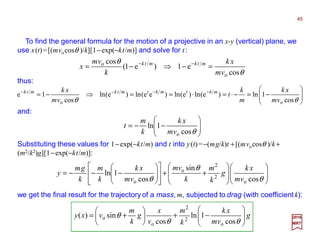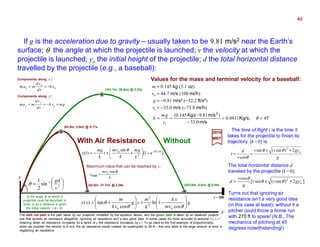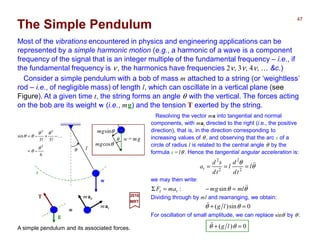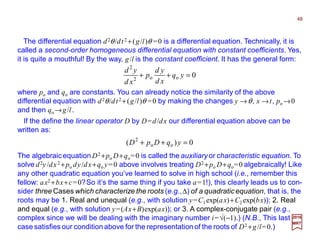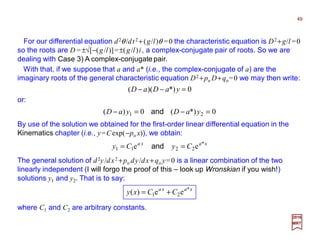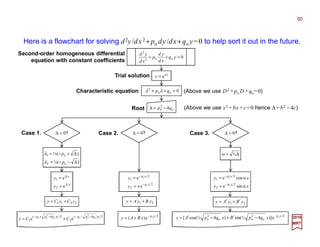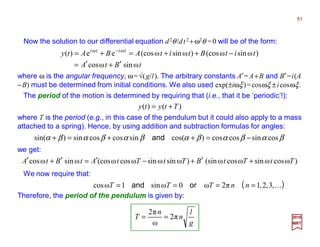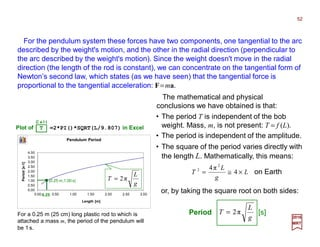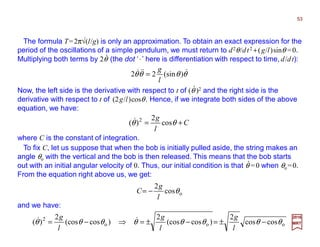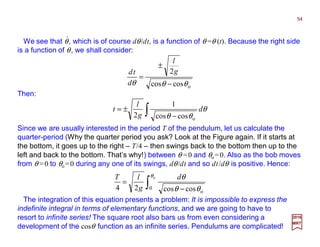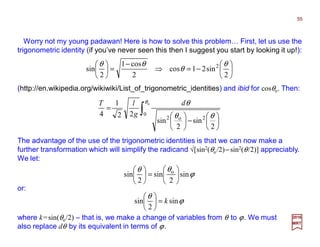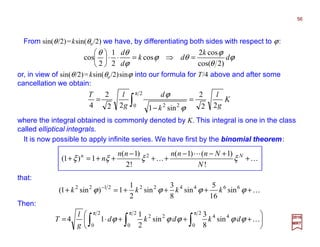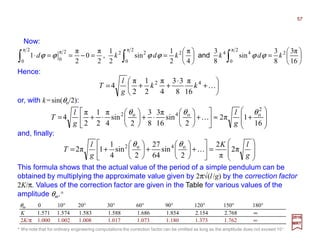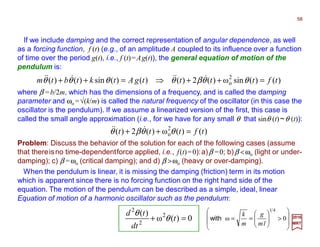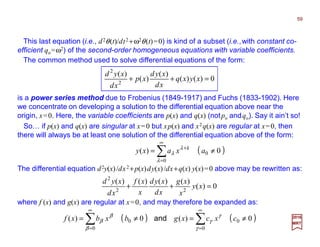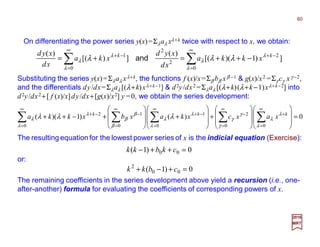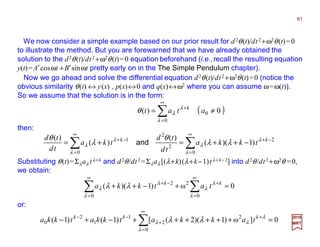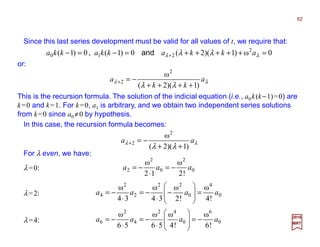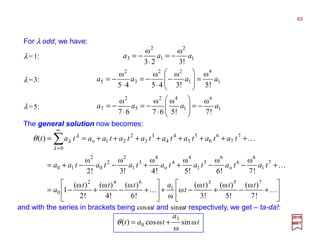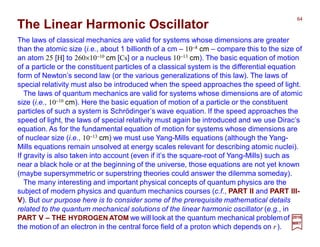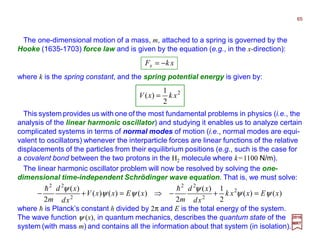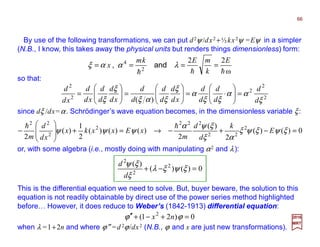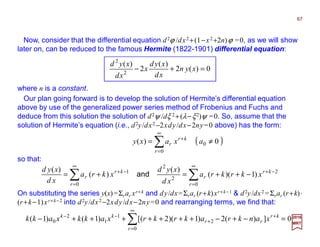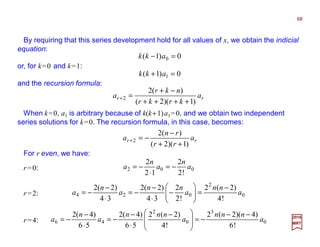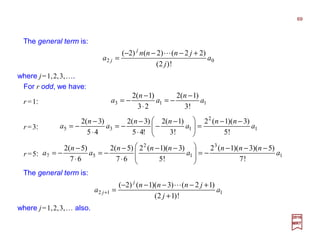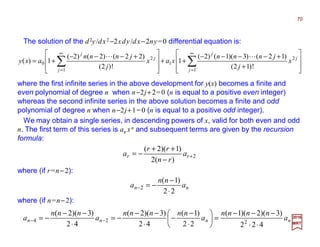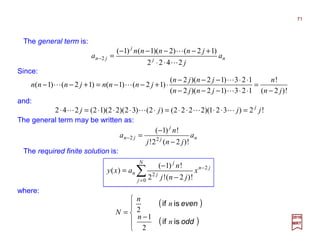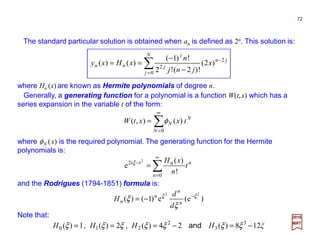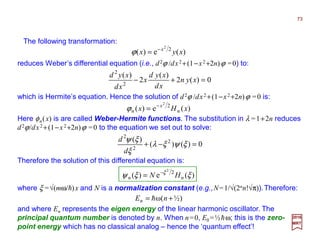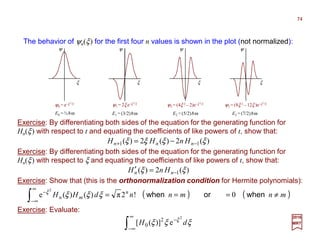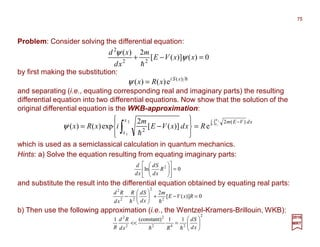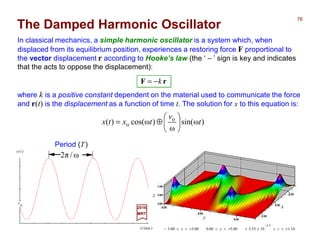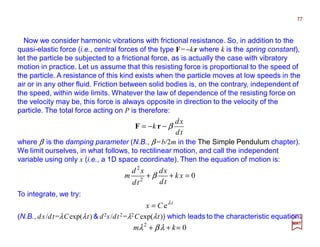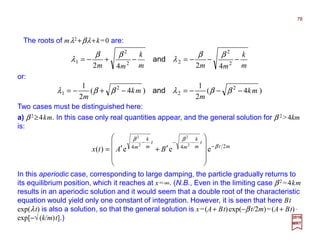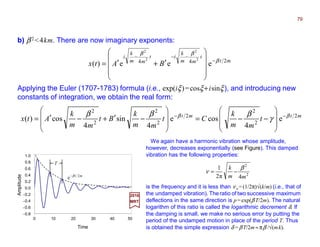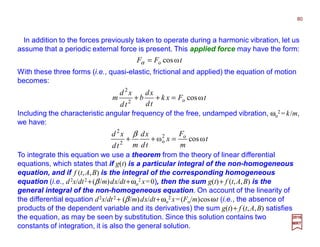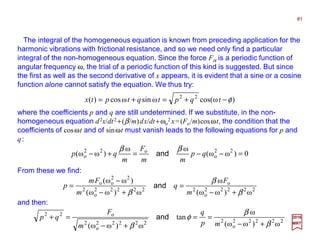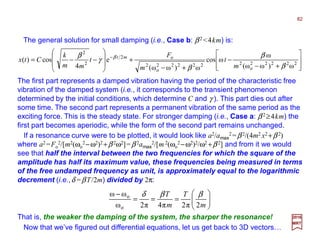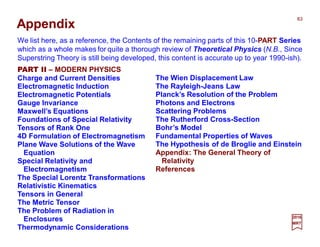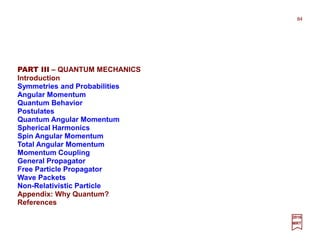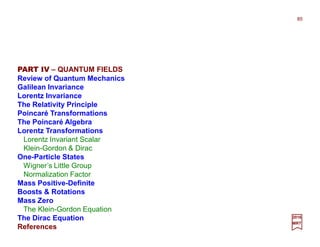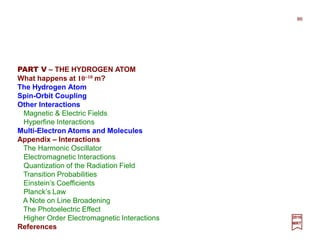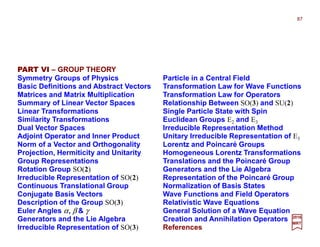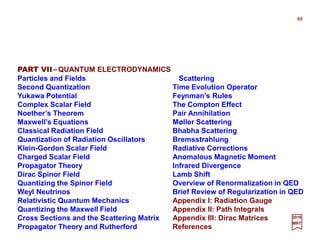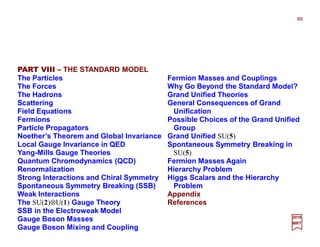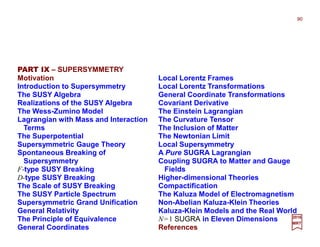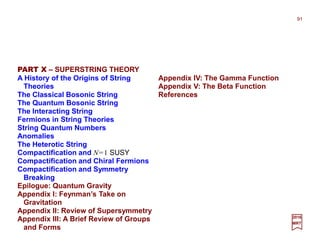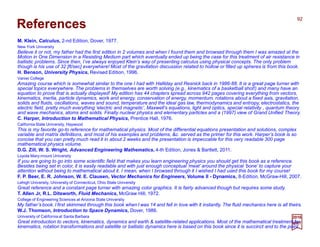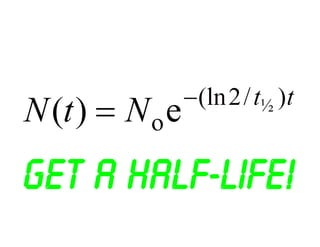1 de 94

### PART I.2 - Physical Mathematics

• 1. BAAABAAA XQQPQQ βαβαµ µ βαβα εδσ == },{2},{ and&& From First Principles PART I – PHYSICAL MATHEMATICS January 2017 – R4.2 Maurice R. TREMBLAY BAAABAAA XQQPQQ βαβαµ µ βαβα εδσ == },{2},{ and&& Chapter 2
• 2. Contents PART I – PHYSICAL MATHEMATICS Useful Mathematics and Infinite Series Determinants, Minors and Cofactors Scalars, Vectors, Rules and Products Direction Cosines and Unit Vectors Non-uniform Acceleration Kinematics of a Basketball Shot Newton’s Laws Moment of a Vector Gravitational Attraction Finite Rotations Trajectory of a Projectile with Air Resistance The Simple Pendulum The Linear Harmonic Oscillator The Damped Harmonic Oscillator General Path Rules Vector Calculus Fluid Mechanics Generalized Coordinates 2016 MRT The Line Integral Vector Theorems Calculus of Variations Gravitational Potential Kinematics of Particles Motion Under a Central Force Particle Dynamics and Orbits Space Vehicle Dynamics Complex Functions Derivative of a Complex Function Contour Integrals Cauchy’s Integral Formula Calculus of Residues Fourier Series and Fourier Transforms Transforms of Derivatives Matrix Operations Rotation Transformations Space Vehicle Motion Appendix 2
• 3. Mass m is a property possessed by all material bodies. Classically, it is a property which describes the effort necessary in giving the body a change in motion. 2016 MRT Newton’s Laws aF m= Newton’s first law is a special case of the second law when F=0. It states that if no force acts on the particle, it will remain at rest or continue to move in a straight line with constant velocity. The equilibrium concept in statics is based on the first law, and the converse of the above statement requires that the resultant of all forces acting on a particle in equilibrium must be zero. The extension of Newton’s laws to a group of particles necessarily involves the action between particles. Actual bodies can be viewed as a group of particles, and to deduce the behavior of such bodies, he introduced Newton’s third law which states: For every action, there is an equal and opposite reaction. Thus if particle 1 exerts a force f12 on particle 2, particle 2 must exert a force f21 on particle 1, where f12 =−f21. 3 Its precise definition is embodied in Newton’s second law, which states that: For a given particle we can write F1/a1 =F2/a2 =F3/a3 =constant where F and a are in consistent set of units. The ratio F/a which is found to be constant for any given particle, is a property of the particle, which is designated as mass, labelled by m. We can therefore write Newton’s second law as: A particle acted upon by a force F will move with an acceleration a proportional to and in the direction of the force; the ratio F/a being constant for any particle.
• 4. tav = 0 1 s 2 s 3 s 4 s 5 s 6 s 0 5 m/s 7.5 m/s 10 m/s 12.5 m/s Speed v(t): Time t: 2.5 F 2 2 1 tas= 0 5 m 11.25 m 20 m 31.25 m 45 m Distance s(t): 1.25 When a constant force F is applied to a mass m it will move with constant acceleration a. 0 2.5 m/s2 Acceleration a=|a|: 2 m/s2.5=a2.5 m/s22.5 m/s22.5 m/s22.5 fkBut if a friction force fk (=µN) is present (i.e., µ ≠ 0) it will oppose the acceleration. N =+mg Nµ µ 40≅ = gmfk 1 s 2 s 3 s 4 s 5 s … s Imagine that a mass m of 4 Kg (4⋅1000 g) is being pushed by a constant force F of 10 Newtons [N]. a) Calculate the uniform acceleration a of the mass m over a distance of 45 meters [m]. b) Represent the motion using a strobe graph in 1-second [s] time intervals and show the various distances s(t) and velocities v(t) corresponding to these time t intervals. Assume that there is no friction between the block and the surface (µ=0): Imagine a 4 Kg block of ice sliding on ice! 2 2 m/s Kg m/sKg Kg Newtons 5.2 4 10 4 10 = ⋅ ==⇒== a m a F a In this case, Newton’s 2nd law is simply F=ma. Dividing this equation by m on both side of the equality sign, we get: Every1s, astrobe ‘ ’ captures the motion of a 4Kg mass under an applied 10N ‘constant’ force… 2016 MRT The acceleration increases by 2.5 m/s (constantly) every second – 2.5 m/s /s! The acceleration due to gravity g is 9.8 m/s /s! 4 TAPE
• 5. M M m h d T Mg +a T +a (1) (2) As an application of an accelerated motion consider Atwood’s machine. It was an apparatus that allowed a direct measurement of Newton’s second law. It could also be used to measure the gravitational constant g (we’ll use m/s2). Two equal blocks of mass M hang on either side of a pulley (see Figure). A small square rider of mass m is placed on one block. When the block is released, it accelerates for a distance h until the rider is caught by a ring that allows the block to pass. From then on the system moves at a constant speed which is measured by timing the fall through a distance d. Show that: 2016 MRT Decomposing the vertical components of the net force: 2 2 2 2 1 2 2 2 )2( th d m mM thm dmM g ⋅⋅ + = + = maMamgMgTF MaMgTMaMgTF y y −−=−−+= +=⇒+=−+= ∑ ∑ (2) (1) and finally for h to d, d =vt which implies v= d/t so we get g ! From 0 to h, v2 =+2ah which implies that a=v2/2h and: g h v m mM =      + 2 2 2 maMamgMgMaMg −−+=+ With T from Eq. (1) we put it into Eq. (2) to obtain: An Atwood machine. where t is the time moved at constant speed. In the lab, one only has to find this time t! (1) (2) 5 ga m mM gmamaM =      + ⇒=+ 2 2 so that:
• 6. mg y Ncosθ N θ θ Nsinθ x fs As an application of a friction force let us consider the scenario depicted in the Figure. A car travels in a horizontal circle around a curve of radius R banked at an angle θ to the horizontal. If µs is the coefficient ofstatic friction, but assuming that µs tanθ <1 and that aR = (v2/R)êR, show that the maximum speed possible without sliding sideways is: 2016 MRT A car is traveling on a banked road at a constant speed v. We start by decomposing the components of the net force: 0sincos 0cossin =−−+= =−−−= ∑ ∑ θθ θθ cy csx FgmNF FgmfF From Eq. (1) above, we get since fs =µs N :     → → =⇔        − + = 0 0tan tan1 tan θµ µθ θµ θµ if if maxmax gR gR vgRv s s s s aR θ θµ θ θ cos sin cos sin gmNgmf F ss c + = + = whereas from Eq. (2) above, we obtain: θθ sincos cFgmN += Thus: θθµµ θ θµθµ tantan cos sincos gmFgm Fgm F css css c ++= + = and finally with Fc =maR =mvmax 2/R and we get vmax above.         − + =⇒+=− θµ θµ θµθµ tan1 tan )tan()tan1( s s cssc gmFgmF and then: (1) (2) 6
• 7. Newton’s laws of motion were formulated for a single particle. If the mass m of a particle is multiplied by its velocity v, the resulting product is called the linear momentum: 2016 MRT vp m= The velocity v here is measured with respect to an inertial frame of reference so that, if the position of the particle is defined by its displacement vector r, the velocity is v=dr/dt. Newton’s second law states that: The time rate of change of momentum is equal to the force producing it, and this change takes place in the direction in which the force acts: p p F &== td d where the dot ‘⋅⋅⋅⋅’ is shorthand for d/dt. With m constant, this equation can also be written: rvF rrvv F &&& mm td d m td d td d m td d m td md ===      === or2 2 )( Newton’s first law, which forms the basis for statics, is a special case of the second law when the force F is zero. It states that: If the forces acting on a particle balance to give a resultant force of zero (i.e., F=p=0), the particle remains at rest (i.e., p=0), or continues to move in a straight line with constant velocity or momentum (i.e., p=constant). ⋅⋅⋅⋅ 7
• 8. mm mm Impact (e=1) V1 V2> v1 v2< If the force F is multiplied by an infinitesimal amount of time dt and integrated, we get: 1212 )(2 1 2 1 2 1 2 1 2 1 vvvvvv vp F mmmmdmtd td d mtd td d td t t t t t t t t t t −=−===== ∫∫∫∫ The time integral on the left side of the equation is called the impulse of the force, so that the above equation states that: The change in momentum of a particle is equal to the impulse of the force acting on the particle. 2016 MRT Momentum before impact is equal to momentum after impact. When no energy is dissipated, the impact is elastic and e= 1 (∆V = ∆v), whereas for the completely plastic impact the relaxation impulse is zero and e =0. In general e depends on the material, shape and the velocities of the two bodies. When two bodies collide, a large force F(t) acts for a short time, and the impulse ∫Fdt exerted on the two bodies must equal and opposite according to Newton’s third law. Since impulse is equal to the change in momentum, for the two bodies considered together as a system, the impulses of collision cancel each other. Thus the change in momentum of the system is zero, and the momentum before impact must equal the momentum after impact. Energy however, is generally dissipated during impact, in which case the impulse during relaxation is less than the impulse during compression. ( ) ( ) approachofVelocity separationofVelocity ncompressio relaxation = ∆ ∆ = − − == ∫ ∫ V v VV vv F F 12 12 td td e The sequence of events is illustrated for e=1 in the Figure. 8 For central impact we let this ratio be e, the coefficient of restitution, and it can be shown that e is also expressible in terms of the velocities as:
• 9. The moment about an arbitrary point O of the momentum p=mv=mR of a particle is: RrprL &m×××××××× ==o where R is the absolute velocity of m and r is drawn from O to P as shown in the Figure (a moment ∆t later, the particle is at P′). Differentiating the equation above, we obtain: Substituting R=Ro ++++r and noting that r××××r=0, this equation becomes: ⋅⋅⋅⋅ ⋅⋅⋅⋅ 2016 MRT Moment about O of momentum mR is r××××mR. ⋅⋅⋅⋅⋅⋅⋅⋅ Moment of a Vector RrRr pr L &&&&& mm td d ××××++++×××× ×××× == )( o ⋅⋅⋅⋅ ⋅⋅⋅⋅ ⋅⋅⋅⋅ ⋅⋅⋅⋅ ⋅⋅⋅⋅ rRRrL &&&&& mm ××××−−−−×××× oo = To establish the relationship between Lo and the moment Mo of the force F=mR (or sum ΣiFi) acting on m, we have: ⋅⋅⋅⋅ ⋅⋅⋅⋅⋅⋅⋅⋅ rRrrrRrRrM mm td d mm ××××−−−−××××++++×××××××× ooo )()( &&&&&&&&& === Substituting Mo =r××××mR into Lo = r ×××× mR– Ro ××××mr above, we can also write: ⋅⋅⋅⋅⋅⋅⋅⋅ ⋅⋅⋅⋅ ⋅⋅⋅⋅ ⋅⋅⋅⋅⋅⋅⋅⋅⋅⋅⋅⋅ rRLM &&& m××××++++ ooo = Generating the moment vector Mo on a diagram is hard to visualize in terms of this sum of the time rate of change of Lo, Lo, and the cross product of the time rate of change of both Roand mr, Ro ××××mr, at the same time. In the Space Vehicle Motion chapter, a physical description using angular rotations and moments of inertia will help us to better visualize the moment Mo in the case of the thrust misalignment of a rocket! ⋅⋅⋅⋅ ⋅⋅⋅⋅ ⋅⋅⋅⋅ 9 y x z R m Ro r O R ⋅ y x z R m Ro r Lo Lo R ⋅ t t + ∆t s s P P′ O′ Lo ⋅⋅⋅⋅ r⋅⋅⋅⋅ Ro ⋅⋅⋅⋅ r⋅⋅⋅⋅ Ro ⋅⋅⋅⋅ m m Mo Lo ⋅⋅⋅⋅ ⋅⋅⋅⋅ Ro ××××mr⋅⋅⋅⋅ Ro ××××r
• 10. Several conclusions can be drawn now: oo LM &= 2. If point O is moving with constant velocity, Ro =0, and: 1. If point O is fixed in space, then Ro =Ro =0 and r=R, which results in the simplified equation: 3. If either Ro or r or Ro and r are parallel, again the simplified equations are valid; 4. If the system consists of more than one mass, labelled by i=1,2,… ,n, the second term of the equation Mo =d(r××××mr)/dt–Ro ××××mr obtained earlier becomes −Ro ××××Σimiri which is zero (Σimiri=0) when the point O coincides with the center of mass. The moment equation is then the same as in Case 2). ⋅⋅⋅⋅ ⋅⋅⋅⋅⋅⋅⋅⋅ ⋅⋅⋅⋅ ⋅⋅⋅⋅ ⋅⋅⋅⋅⋅⋅⋅⋅ which states that the moment is equal to the rate of change of the apparent moment of momentum (or angular momentum) expressed in terms of the relative velocity r; Fr rr r rrrM ×××× ×××××××××××× = === && & & m td d mm td d )(o ⋅⋅⋅⋅ ⋅⋅⋅⋅⋅⋅⋅⋅ ⋅⋅⋅⋅ ⋅⋅⋅⋅ ⋅⋅⋅⋅ ⋅⋅⋅⋅⋅⋅⋅⋅ ⋅⋅⋅⋅⋅⋅⋅⋅ 2016 MRT 10
• 11. [9,6,−2] A(1,−3,2) P(4,−1,7) x y z r F Mo Let’s get right into the physics of the cross product: the Moment of a Vector! This time we will use the concept of the force vector to visualize the moment of a vector... The moment Mo will be independent of where r terminates on F or on its line of action. nFrM ˆ)sin(o ϕrF== ×××× 2016 MRT The moment of force vector Mo normal to plane of position vector r and force vector F is r××××F. ˆ Exercise: A force of 5 lb which acts through the point P(4,−1,7) in the direction of the vector [9,6,−2]. Find the moment of this force about the point A(1,−3,2). We consider a vector F and any point O in 3D space. If we draw a vector r from O to any point on F or on the line of action of F (see Figure) and form the cross product with r as the first vector, then the result will be a moment of force Mo about the axis through O in a direction perpendicular to the plane containing r and F, the direction of which is indicated by the unit vector n: 11 Mo nˆ r ϕ In a plane (e.g., 8½×11 paper) The vector Mo comes out of front of page. F O Solution: The position vector is r =(4 −1)i ++++(−1 +3)j ++++ (7−2)k = 3i ++++2j ++++ 5k and |r|=√(32 +22+52)= √(38)≈6 [ft] while the force vector is F=(9− 4)i ++++(6 −1)j ++++ (−2 −7)k =5i +5j−−−− 9k and |F|=5 [lb]. The formula for the moment is Mo = (|r||F|sinϕ )n but ϕ is unknown so we will have to compute Mo =r ×××× F= =(−18− 25)i −−−− (−27 −25)j ++++ (15 −10)k =−43i −−−−52j ++++ 5k and |Mo |=√(422 + 522+52)= √(4578)≈68 [lb⋅ft]. Since r •F=3⋅5 +2⋅5 +5⋅−9 =−20 = |r||F|cosϕ =6⋅5 cosϕ gives cosϕ =−20/30 so we get ϕ =132°. 955 523 ˆˆˆ − kji ˆ ˆ ˆ ˆ ˆ ˆ ˆ ˆ ˆ ˆ ˆ ˆ ˆ ˆ ˆ ˆ ˆ ˆ ˆ
• 12. l=30 cm Biceps (Tension T) 4 cm θ = 10° d +ττττ wArm wBall FH FV T +g Humerus Radius Ulnaττττ d l As an application of a moment of force (here torque ττττ is used in a context of an applied weight w=−mg)letus considerthe scenario depicted in the Figure which shows a diagram of an arm and the biceps muscle. The muscle is attached at a point about 4 cm from the socket which acts as a pivot point. If a weight of 50 N is held in the hand, what is the ten- sion in the muscle? (Assume that the forearm is a horizontal uniform rod of weight 15 N and lengthl=30cm andthattheforce exertedby the muscle acts at10° to the vertical.) The Figure (ττττ out of page) shows the forces acting on the forearm, where FH and FV are the horizontal and vertical components of the force exerted by the pivot of the forearm. 2016 MRT Since the muscle is close to the pivot point, it must exert a force much larger than the weight of the ball (i.e., using wBall =mg: 50 N =m⋅ 9.8m/s2 ⇒ m ≅ 5 kg). By taking torques about the pivot we avoid the force exer- ted by the pivot. Thus, using the components of the torque ττττ =r ××××F (i.e., with its magnitude being |ττττ|=|F||r|sinθ for d, l and T instead) explicitly under static equilibrium conditions: 0)1()3.0()50()1( 2 3.0 )15()1()04.0()10cos( 090sin90sin 2 90sincos =⋅⋅−⋅      ⋅−⋅⋅= =⋅⋅−⋅⋅−⋅⋅+=∑ mN m Nm BallArm o ooo T lw l wdT θτ Thus, T = |T|=438 N, which is considerably greater than the weight wBall (i.e., 50 N or ∼10×). As for the forces FH and FV : V y H x wwTF TF F F ==−−= −−= === = ∑ ∑ NNNN NN BallArm 3665015)10)(cos438( cos 76)10)(sin438( sin o o θ θ 0wlw l Tdτ ==∑ BallArm ××××−−−−××××−−−−××××++++ 2 12 Humerus
• 13. m m ev N θ ⋅⋅⋅⋅ l CM Problem: A dumbbell idealized by two masses on a stiff, weightless rod of length l is dropped without rotation, and the left mass strikes a ledge with velocity v. Assume a coefficient of restitution to be e (e.g., e=1 collide elastically, e<1 collide inelastically and for e=0, objects effectively stop at the collision, not bouncing at all). Determine the angular rotation of the dumbbell immediately after the impact. Solution: The dumbbell immediately after impact is as shown. ⋅⋅⋅⋅ )2( 2 2 mv l vemtdN −−      −=∫ θ& where N is the normal force and the change about the angular momentum about the center of mass is equal to the moment about the center of mass of the impulse ∫Ndt and is: θ& 2 2 2 2       =∫ l mtdN l Eliminating the impulse integral, the angular velocity immediately after the impact is: )1( e l v +=θ& 2016 MRT The velocity vCM of the center of mass immediately after the impact is ev−(l/2)θ, and the change in the linear momentum mv is: 13
• 14. The basic concepts of mechanics are space, time, and mass(or force),which are more or less understood intuitively by most beginning students. To be useful for quantitative analysis, however, these notions must be viewed as mathematical concepts related to each other by fundamental laws. Such a formulation was introduced by Newton in his three laws of motion which have become the foundation for the classical mechanics or Newtonian mechanics. Although the likes of Le Verrier or Herschel would be jealous! Newton’s laws were formulated for a single particle and our notion of a particle is that of a material body of infinitely small dimensions. In mechanics we can expand this notion to include any material body whose dimensions are small in comparison with distances or lengths involved in defining its position or motion. Thus planets can be considered to be particles when their position in the solar system is taken under consideration. Gravitational Attraction In defining the position of a particle in space, a frame of reference is necessary. For this purpose, three perpendicular lines intersecting at a common point called the origin are adequate. The position of the particle can then be defined in terms of distances along these lines. Each component is squared then summed and the result square- rooted to give a position vector. To describe the motion of a particle, the concept of time is required. By noting the position of the particle as a function of time, its motion (e.g., described by its displacement, velocity, and acceleration) is completely defined. Force is known to us intuitively as a push or a pull which is represented by the action of one body on another, exerted by contact or through a distance as in the case of the gravitational (or electromagnetic) force. To describe a force, it is necessary to know its magnitude, its direction, and its point of application. 2016 MRT 14
• 15. PT′ r R Q T t m Q′ 1444442444443 D R′ The most interesting case of gravitation attraction is, of course, the attraction exerted by the Earth. We shall take the Earth to be a solid sphere of mass M⊕ per unit volume (and surface radius Rs) and calculate the force that the sphere exerts on a mass m. The calculation of the gravitational attraction that a thin spherical shell exerts poses a problem in itself. Suppose that an object of mass m is located at point P (see Figure) which is D units from the center O of the shell. The shell has a thickness that we shall denote by t. The parts of the shell are all at different distances from P, and so we cannot apply the law of gravitation (i.e., F=GmM⊕/r2 below) at once to the shell and the mass m. The force which the Earth exerts on any object at any distance from the Earth’s center is given by the famous axiom of physics known as Newton’s law of gravitation. This law states that any two objects in the universe attract each other, and the force with which each attracts the other is given quantitatively by the formula: In this formula G =6.674×10−11 N⋅(m/kg)2 is the gravitational constant; it is the same number no matter what the masses involved and no matter what the distance r between them. The quantities m and M are the masses of the two objects.         === ⊕ 2 s ss2 R MG ggm r Mm GF if An object of mass m is located at point P. 2016 MRT 15 We can regard the shell as composed of small zones. Thus the circles QR and Q′R′ bound a zone on the outer sphere of the shell, and if we take this zone to have the thick- ness t of the shell, we may regard the shell as composed of a number of such zones. O
• 16. O P r R′ R Q′ Q T m t f T′ U A U′ θ dθ ∠OPQ a dV D r aD PQ OA PQ AP OPQ θcos )cos( − = − = =∠ Let us consider, then, the attraction exerted by any one zone on the mass m. We think of the zone (see Figure) as made up of equal little pieces such as U′U TT′, each of which pulls the mass m. What we should seek is the component PO that each piece of the zone exerts. These components can all be added to obtain the attraction caused by the zone because they are in the same direction. The little pieces of any one zone all make about the same angle with PO, namely the angle∠OPQ, and each piece is the same distance r from the mass m. Hence the compo- nent of the force f along PO that each piece of the zone exerts is the same. If we let ∆V be the volume of each piece of the zone, then M∆V is the mass of the piece (we will also keep M as unspecified from now on). The force exerted by this piece along PO is: The sum of all these components is: where θ is the angle shown in the Figure. )cos(2 OPQ r mMG dV ∠⋅ An object of mass m is located at point P. 2016 MRT )cos( )( 2 OPQ r mMVG ∠ ∆ where V is the volume of the zone. This volume is approximately t times the area of the zone (i.e., dV=t⋅dA). The area is approximately the circumference of the circle QR multiplied by the arc length QQ1. This area is: θθθ daadaQAAd sinπ2π2 =⋅⋅= 16 OP
• 17. Then the component along PO of the force f exerted by the entire zone is: since dV=t⋅dA and dA=2πasinθadθ. If we denote this component of f by f , we get: r aD PQ OAOP PQ AP OPQ θcos )cos( − = − ==∠ 2016 MRT )cos(sinπ2)cos()cos( 222 OPQ r mGM daatOPQ r mGM AdtOPQ r mGM Vd ∠⋅⋅=∠⋅⋅=∠⋅ θθ Let us now find an expression for ∠OPQ in this last equation. We have from the previous Figure that: )cos( sinπ2 2 2 OPQ r datmGM f ∠ ⋅ = θθ where D is the distance from the center of the sphere, O, to the mass m at P. Then: r aD r datmGM f θθθ cossinπ2 2 2 − ⋅ ⋅ = This last equation contains two variables (i.e., θ and r) and we must eliminate one of these to obtain the integrand of our ultimate definite integral. Fortunately, the law of cosines, c2 =a2 +b2 −2abcosC, applied to triangle OPQ furnishes us with the relation: θcos2222 aDDar −+= This equation defines r as a function of θ. 17 ab c A B C ra D θ≡ →
• 18. By differentiating r2 =a2 +D2 +2aDcosθ with respect to θ, we obtain: To eliminate the remaining term in θ, we again use r2 =a2 +D2 +2aDcosθ. From it we have that: 2016 MRT θθ dDardr sin= The use of this relation to replace asinθ in our last equation for f yields: r aD rD rdratMmG f θcosπ2 2 − ⋅ ⋅ = θθ cos 2 cos22 222 2222 aD D aDr DaDaDr −= −+ ⇒−=−+ If we use this result in our result for f above, we have: rd r aDr D atGmM rf 2 222 2 π )( −+ ⋅ ⋅ = The quantity f(r) is the attractive force along PO which is due to the single zone formed by QRR′Q′. To obtain the attractive force due to the entire shell, we must first sum all elements of the form f(r) above one for each zone, and then let the number of zones become infinite while the width dr (or ∆r) of each zone approaches 0. If we visualize the various zones that fill out the spherical shell in the first Figure, we see that r will range from the value PT for the nearest zone to P to PT′ for the farthest zone from P. Then r ranges (i.e., the bounds of integration) from D−a to D+a. 18
• 20. O P mx D dx R The force F is exactly what one would obtain by regarding the mass of the shell as concentrated at the center of the shell and by applying the law of gravitation to the two masses m and Mt⋅πa2 separated by the distance D. In other words, a spherical shell acts, insofar as its gravitational attraction on an external mass is concerned, as though the mass of the shell were concentrated at the center (e.g., you can call that Gauss’ theorem for gravity – technically expressed as ∫∫S g•dS=−4πGM with g=−∇∇∇∇φ). We are now able to consider the gravitational force that a solid sphere of radius R exerts on an external particle (point mass) of mass m. We shall regard the sphere as a sum of n spherical shells (see Figure). However, in place of the notation t for the thickness of each shell we shall use the quantity ∆D or dx. An object of mass m is located at point P. 2016 MRT We did already show that the gravitational attraction of a thin shell may be computed by regarding the mass of the shell as concentrated at its center. Hence, with F= GmMt⋅4πa2/D2, the force that the typical shell exerts on the particle of mass m is: xd D xGmM xdxF ⋅ ⋅ =⋅ 2 2 π4 )( where D is the distance OP, that is, the distance from the center of the location of the particle. The calculations of the gravitational force exerted by the Earth is not really disposed of by all the previous or following text, for the Earth is not a sphere but an oblate spheroid, that is, one type of ellipsoid and to, say, calculate the moon’s motion the elliptical shape must also be taken into account! 20
• 21. To obtain the gravitational force exerted by the solid sphere, we sum the forces exerted by the n concentric shells while the width of each shell approaches 0. Such a limit of a sequence of sums is a definite integral. To write down the definite integral, we need only the additional knowledge that x varies from O (i.e., 0) to R. If we let F be the force exerted by the solid sphere, then: or: 2016 MRT ∫ ⋅ = R xd D xGmM F 0 2 2 π4         =        ⋅ = ⋅ = ∫ 3 π4 3 π4π4 )( 3 2 3 20 2 2 MR D GmR D GmM xdx D GmM RF R This result has a very simple physical interpretation.The quantity 4πR3M/3 is the mass of the entire sphere. Because D is the distance from the center of the sphere to the particle at P, we see that the whole sphere acts on the external particle as though the sphere’s mass were concentrated at the center. The usual law of gravitation applies to the two masses with the distance between them the distance from the center of the sphere to P. The question also arises as to how does the Earth act on a particle inside (e.g., ass- ume that a tunnel is dug through the Earth to reach Australia)? If we were to make the calculation we would find that a thin spherical shell exerts no attractive force on any point mass or particle inside the shell. This also applies to shells of any thickness, i.e., even a shell of finite thickness exerts no force on a particle interior to it! 21
• 22. From his interest in astronomy, and from the calculation outlined above to show that all the mass is as if it were concentrated at the center, Newton formulated the law of gravitation between two particles. The law states that any two particles attract one another with a force of magnitude: 2016 MRT 2 21 r mm GF = where m1 and m2 are the masses of the particles 1 and 2, r is the distance between them, and G is the universal constant of gravitation. gmw = and by comparison with the previous equation, we arrive at the result g=GM⊕/R⊕ 2. We find then that the acceleration of gravity g will vary inversely with R⊕ 2. Since m is a pro- perty of the body which is fixed, its weight will then vary with g and R⊕, and so we find a given body weighing somewhat different amount at different places on the Earth’s surface. At the Earth’s surface g=gs is nearly 32 ft/sec2 [CGS] or 10 m/s2 [MKS]. 22 Application of this law to a particle on the Earth’s surface gives us an understanding of the relationship existing between mass and weight. Letting m1 = M⊕ and m2 =m be the mass of the Earth and that of another body as the Earth’s surface, a distance equal to the radium r=R⊕ from the center, the attraction of the Earth on the body, which is called the weight, w, is given by interpreting the above equation as w=m(GM⊕/R⊕ 2)! If this force is not opposed to a support of some kind, the body will fall toward the center of the Earth with an acceleration g. Thus from Newton’s second law, we can write:
• 23. We know that the force of gravity varies from place to place on the surface of Earth. There are two reasons behind this variation: the shape of Earth and the rotation of Earth. The Earth is not a perfect sphere, but bulges at the equator. Therefore if a body is taken from pole to equator its distance from the centre of the Earth will change. Consequently, the gravitational force also varies, although totally imperceptible to us on any given day! 2016 MRT 2s ⊕ ⊕ = R M Gg Consider the variation of gs when a body moves distance upward or downward from the surface of Earth. Let gs be the value of acceleration due to gravity at the surface of Earth and gh at a height h above the surface of Earth. If the Earth is considered as a sphere of homogeneous composition, then gs at any point on the surface of the Earth is: 23 Now we will determine the value of gs at a distance R⊕ +h from the center of the Earth. The acceleration due to gravity is: 2 )( hR M Ggh + = ⊕ ⊕ Then: 2 2 22 2 2 2 s )()( )(         + = + =⋅ + = + = ⊕ ⊕ ⊕ ⊕ ⊕ ⊕ ⊕ ⊕ ⊕ ⊕ ⊕ ⊕ hR R hR R M R hR M R M G hR M G g gh
• 24. Pushing now to simplify things we get by dividing numerator and denominator by R⊕: 2016 MRT Using the binomial theorem: 24 and considering the replacement of x and n by h/R⊕ and −2, respectively, to obtain: which means that the value of g at a height h above the surface of Earth, gh, is 1−2h/R⊕ times that of gs, the acceleration due to gravity at the surface of Earth (i.e, R⊕): 222 s 1 1 1 − ⊕⊕⊕ ⊕         +=        + =        + = R h RhhR R g gh xnx n +≅+ 1)1( ⊕ −≅ R h g gh 2 1 s s 2 1)( g R h hggh         −≅= ⊕ If the height h above the surface of Earth is about half the Earth’s radius, ½R⊕, away then the value of Earth’s gravity at that height is g½R⊕ =(1−1)gs=0 (i.e., total weightlessness). At half that distance, ¼R⊕, you would only feel half your weight since g¼R⊕ =(1−½)gs=½gs.
• 25. gh R⊕ gs h • h R(φ) Space Shuttle Orbit ti tf = ti + T • • •O gs is the value of g at Earth’s surface (9.807 m/s/s or m/s2), R⊕ is the radius of the Earth (6.38×106 m), h is the altitude above Earth [m], gh is the value of g at altitude h [m/s/s]. The percentage difference in radii is 1.0025%! These different values for the Earth’s radius is a characteristic of the Earth’s oblateness (i.e., the Earth is an oblate spheroid!) The Earth’s equatorial radius of curvature in the ‘meridian’ is b2/a or 6335.437 km. The Earth’s ‘polar’ radius of curvature is a2/b or 6399.592 km. Difference is ∆ ≅ 1%! The Earth’s radius at a geodetic latitude,φ, is given by the radius of the Earth formula: = gsgh 22 2222 )sin()cos( )sin()cos( )( φφ φφ φ ba ba RR + + ==⊕ 2016 MRT 2         +⊕ ⊕ hR R 25 The plot of: in Excel shows that gh will decrease linearly with altitude (e.g., The space shuttle reaches an altitude of 300 km). C e l l gh =gs*(R/(R+h))^2
• 26. So far we have avoided one very important question regarding the frame of reference used in the measurement of the motion. 2016 MRT 26 Newton assumed that there was a frame of reference whose absolute motion was zero. He considered such an inertial frame fixed relative to the stars to be one of absolute zero motion, and his laws of motion to be valid when referred to such a reference. Controversies regarding the existence of such reference frames of absolute zero motion led to the formulation of the theories of relativity for which Newtonian mechanics is a special – or ‘limited’ or even better coined as the ‘effective limit’ – case. In arriving at the concept of weight, it was necessary to measure gs relative to the surface of the Earth which is not at rest. Thus the acceleration of the Earth’s surface due to its rotation must be accounted for in a more exact analysis. For many problems this error is insignificant, in which case the Earth’s surface will be found to be an adequate reference. There are other problems, however, such as navigation for space flight, where the Earth’s surface cannot be considered stationary. In general, problems in space dynamics are involved with rotating and accelerating coordinates, and the subject of relative motion and transformation of coordinate play an important role.
• 27. Finite angular rotations of a rigid body do not obey the law of composition (which states that the sum of two vectors is represented by the diagonal of a parallelogram formed by the two vectors as sides). It has magnitude equal to the angles of rotation, which can be directed along the axis of rotation. However, two such rotations along different axes are not commutative and will not add up to the diagonal of a parallelogram (e.g., Pick up an iPhone and lay it flat in your right hand so that your fingers can wrap around it. Rotate it 90° about the 1- and 3-axes with your left hand. You need to hold it up sideways! Then repeat the procedure in the reverse order. You will need to hold it up facing you! Thus finite angular rotations are shown to be non-commutative: 1⋅3≠3⋅1) Infinitesimal rotations however can be shown to be commutative (i.e., R⋅R−R⋅R=0 so here we have 1⋅3=3⋅1⇒1⋅3−3⋅1=0) and to possess all the properties of a vector. 2016 MRT The angular velocity represented by a vector. To show this, consider the displacement of a point P(r) due to two infinitesimal rotations ω1 dt and ω2 dt about any two axes, where ω1 and ω2 are their respective rotational speeds. Let the direction of each of the axes of rotation be indicated by unit vectors ê1 and ê2 as shown in the Figure, and we will perform the rotations in the order 1 and 2, then repeat in the reverse order to examine the final result. Because of the infinitesimal rotation (ω1 dt)ê1, the end of the displacement vector r defining the position P will be displaced to P′ by an amount (see Figure): re ××××11 ˆ)ω( td and the new position is defined by the vector (see Figure): rerr ××××++++ 111 ˆ)ω( td= Finite Rotations ê1 r ω1 r1 O ê2 ê3 ω2 ê1 r ê1×××× r P P (ω1 dt)ê1×××× r 27 P′
• 28. r1 ω2 r2 P ê1 ê2 O ê3 ω1 r ê1 r Next allow the second infinitesimal rotation (ω2dt)ê2, starting from P′, in which case the final position is P(r2) and is defined by the vector r2: reererer rerererrerr ××××××××++++××××++++××××++++ ××××++++××××++++××××++++××××++++ 11222211 11221112212 ˆ)ω(ˆ)ω(ˆ)ω(ˆ)ω( ]ˆ)ω([ˆ)ω(]ˆ)ω([ˆ)ω( tdtdtdtd tdtdtdtd = == 2016 MRT Neglecting the second-order term (i.e., ω1ω2(ê2××××ê1××××r)dt2) above, we arrive at the result: tdtd rrreerr ××××ωωωω++++ωωωω++++××××++++++++ )()ˆωˆω( 2122112 == since ωωωωn=ωnên. If we repeat the operation in the reverse order, we will find the equation for r2 to be identical to the case above, indicating that infinitesimal rotations are commutative (at least to first-order since we neglected the second-order – or quadratic – term.) 28 P′
• 29. ê2R(ϕ)ê2 R(ϕ)ê1 ê1 O ϕ ϕ You can skip this… Consider a system symmetric under a rotation in a plane, around a fixed point O. If we adopt a Cartesian coordinate frame on the plane with ê1 and ê2 as the other normal basis vectors (see Figure). Denoting the rotation through the angle ϕ by R(ϕ), we obtain by elementary geometry (see the Matrix Operations chapter): ϕϕϕϕϕϕ cosˆsinˆˆ)(sinˆcosˆˆ)( 212211 eeeeee ++++++++ −== RR and R(ϕ) has interesting properties such as two rotation operations can be applied in succession, resulting in an equivalent single rotation R(ϕ2)R(ϕ1)=R(ϕ2+ϕ1) (i.e., techni- cally here, R(ϕ1) goes first then R(ϕ2) next) but R(ϕ1)R(ϕ2)≡R(ϕ2)R(ϕ1) which equates to reversing the order of operation of the rotation with no change in the outcome! A rotation by and angle ϕ of two unit vectors ê1 and ê2 in a plane. J is the generator of rotation. The differentiability of R(ϕ ) in ϕ requires that R(dϕ) differs from R(0)= 1 (i.e., the identity – or ‘nothing happens’ or it ‘ends up the same’) by only a quantity of order dϕ which we define by (i2 =−1): JdidR ⋅−= ϕϕ 1)( 2016 MRT where the quantity J is independent of the rotation angle dϕ. Next, consider the rotation R(ϕ +dϕ), which can be evaluated in two ways: ϕ ϕ ϕϕϕϕ ϕϕϕϕϕϕϕϕϕ d Rd dRdR JRidRJdiRdRRdR )( )()( ])([)()1)(()()()( +=+ −+=⋅−==+ Comparing the two equations, we get the differential equation: 0)( )( )( )( =+⇒−= JRi d Rd JRi d Rd ϕ ϕ ϕ ϕ ϕ ϕ with solution R(ϕ )=exp(−i ϕ J) and boundary condition R(0)=1.       − =⇒    −= += ϕϕ ϕϕ ϕ ϕϕϕ ϕϕϕ cossin sincos )( cosˆsinˆˆ)( sinˆcosˆˆ)( 212 211 R R R eee eee ++++ ++++       − −− =             − ==− ϕϕ ϕϕ ϕ ϕ ϕ ϕ ϕ ϕ ϕ ϕ ϕ ϕ ϕ sincos )cos(sin cossin sincos )( )( d d d d d d d d d Rd JRi       − =      + −       − − ϕϕ ϕϕ ϕϕ ϕϕ sincos cossin 0 0 cossin sincos i i i       + − = 0 0 i i JJ istheif generator 29
• 30. vx i vy j −kvy j −kvxi Gravity Velocity v Air Resistance −mg Fair=−kv Below is a free body diagram (not to scale) for a projectile that experiences air resistance and the effects of gravity (the dashed vectors are the x and y components of velocity and air resistance). Here we assume that the air resistance is in the direction opposite of the projectile’s (e.g., baseball) velocity. Trajectory of a Projectile with Air Resistance When the velocity of the projectile is 4 m/s, the air resistance is 7 N. When we double the velocity to 8 m/s, the air resistance doubles to 14 N accordingly. In this case, k=7/4= 1.75 N⋅s/m – i.e., 75% more resistance to travel than without air resistance! We can write that the air resistance is an inverse linear function in the velocityv with k as the drag coefficient (N.B., Atmospheric drag is highlighted below but not considering uncertainties in atmospheric fluctuations, Earth’s oblateness, &c. If we consider only the cross-sectional area A perpendicular to the direction of motion, the dimensionless drag coefficient CD associated with A, and other parameters such as the speed of the mass m relative to the atmosphere va and the atmospheric density ρ at the mass’ altitude): 2016 MRT tCoefficien Ballistic 1 2 1 =   −=−= mg ACD aD vACvkF ρAir ˆ ˆ ˆ ˆ 30
• 31. Neglecting the Magnus effect (i.e., basketball rotation via (16/3)π2rbasketball ρ ωωωω××××v) the formula for calculating the drag force of an object moving through a fluid is thus: 2 2 1 aDD vACF ρ= where ρ is the density of the fluid and A is the area of the ball. If the fluid is air, then for air at sea level we have ρ=1.2 Kg/m3.The coefficient of drag for this shape is CD ~0.5 (i.e., for most spheres for most sports conditions.) For a basketball with v=20 m/s and area A=0.046 m2, we then have FD =½⋅1.2⋅400⋅0.5⋅0.046=5.5 N. The weight of the ball is w=mg=4.5⋅1.31=6 N so this is comparable to drag force. CD is not a fixed number but depends on Reynolds number and surface smoothness. This calculation of drag force is close enough. Given a range R of a ball launched at 45 degrees, from kinematics we have R=vo 2/g where vo is the launch velocity assuming the ball is launched from the height of the basket – i.e., a tall man with jump shot. For a 3 point shot, R=24 feet=7.3 m. Given g=10 m/s2, vo =√(Rg)=√(7.3⋅10)=8.5 m/s. For a 3 point shot, launch velocity in vacuum is 8.5 m/s so the drag force is FD =(8.5/45)2=0.036≥3.6% of its weight. Since the range varies as the square-root of the kinetic energy,√T, and that the drag force is proportional to the loss of total energy, the range is reduced by about 1.8% or 0.13 m (i.e., 13 cm). The basket has an allowance of 0.15/2 m so the loss of range doesn’t cause a clean miss! The players obviously practice shooting at the actual target hoop to get used to the way it looks and to get the feel for the launch velocity from experience. For shorter shots, the change in range is considerably smaller due to the launch velocities which are much smaller than that of the terminal velocity. 2016 MRT 31 2
• 32. Now back to the resistance problem. Here we assume that the air resistance is in the direction opposite of the projectile’s velocity. We can write that the air resistance is an inverse linear function in the velocity v with k as the drag coefficient: because initial assumptions of proportionality implies that the air resistance and the velo- city differ only by a constant arbitrary factor, and that when v is increased by a factor of, say, p, the air resistance increases by a factor of p also. vF k−=Air To derive relationships to represent the motion of the particle, we first apply Newton’s Second Law (i.e., the sum total of all vector forces F=FWeight++++FAir equals the product of the scalar mass m by its vector acceleration a: ΣF=ma=m(axi++++ay j)=0) for both the x and y components: ˆˆ The weight mg term is positive because the value of g is already negative and subtracting it would result in a positive number. Note that acceleration is just the derivative (i.e., infinitesimal rate of change) of velocity with respect to time (i.e., a=dv/dt). 0=+=∑ x x x kv dt dv mF 2016 MRT 32 0=−+=∑ mgkv dt dv mF y y y and
• 33. Solving the first of these equations, mdvx /dt=−kvx, is an elementary problem in solving differential equations that we will show next, starts by re-writing it in the standard form: and we get: where A is an arbitrary constant. The quantity exp[−∫p(x)dx] is referred to as the integrating factor. xdxp y yd yxp xd yd )(0)( −=⇒=+ ∫− =⇒−== ∫∫ xdxp Ayxdxpy y yd )( e)(ln nIntegratio tables We now multiply the original equation dy/dx+p(x) y=q(x) by the inverse of the integrating factor. On multiplying it by exp[+∫p(x)dx] we obtain: ∫∫ =      + xdxpxdxp xqyxp xd yd )()( e)(e)( 2016 MRT We now solve the corresponding homogeneous equation (i.e., with q(x)=0): 33 this differential equation in vx is of the general form dy/dx+p(x) y=q(x) and is called a first-order linear differential equation. Notice the similarity of dy/dx+p(x) y=q(x) with dvx /dt+(k/m)vx=0 by making the changes y →vx, x →t, p(x)→k/m and q(x)→0. 0=+ x x v m k xd vd
• 34. Since: our last equation (i.e., [dy/dx+p(x) y]exp[∫p(x)dx] =q(x)exp[∫p(x)dx]), may be written as: Integrating this equation, we get: or: This is the general solution of the differential equation dy/dx+p(x) y=q(x). where C is an arbitrary constant of integration. Finally, when p(x) is independent of x it is a constant, say po, and the differential equation is dy/dx+po y=0 and its solution becomes y=Cexp(−po ∫dx)=Cexp(−po x). Note this since we will be using it again soon. 2016 MRT ∫∫ = xdxpxdxp xqy xd d )()( e)(]e[ ∫∫∫∫       +=+=⋅ xdxpxdxpxdxpxdxp yxp xd yd xpy xd yd y xd d )()()()( e)(]e)([e]e[ Cxdxqyyd xdxpxdxpxdxp +== ∫∫ ∫∫∫ )()()( e)(e]e[ ∫−∫∫− += ∫ xdxpxdxpxdxp Cxdy )()()( eee )(xq ∫− = xdxp Cy )( e When q(x)=0, the differential equation reduces to dy/dx+p(x) y=0 and it has the solution: 34
• 35. As a simple application let us consider (Jacob) Bernoulli’s (1654-1705) equation: This differential equation is non-linear since it contains a non-linear term in the dependent variable, yn, but it can be reduced to the linear form. To reduce dy/dx+p(x) y= q(x)yn to the linear form, we first make a change of variable. Let: so that: On multiplying dy/dx+p(x) y=q(x)yn by (1−n)y−n, we obtain: which is the linear form that was sought. This differential equation is the required linear form. It can be solved by use of the method just outlined and its solution is (N.B., n>1): 2016 MRT ( )1)()( ≠=+ nyxqyxp xd yd n n yz − = 1 xd yd yn xd zd n− −= )1( )()1()()1( xqnzxpn xd zd −=−+ In terms of the variable z, we write: )()1()()1()1( 1 xqynxpyn xd yd yn nn −=−+− −− ∫−−∫−∫−− +−= ∫ xdxpnxdxpnxdxpn Cxdxqnz )()1()()1()()1( ee)()1(e 35
• 36. Now, I could not pass the chance of discussing a simple application of the first-order linear differential equation – that of radioactive decay. Radioactivity was discovered in 1896 by Becquerel (1852-1908) while working on phosphorescent materials. In the ra- dioactive decay of unstable nuclei, we find that the process is governed by the following differential equation. The time rate of change of N(t) is proportional to decreasing N(t): where N(t) represents the number of parent atoms present at time t, λ is the decay (or disintegration) constant which is characteristic of the particular element involved, and the negative sign is used to indicate that the number of parent atoms decrease with time. where the minus sign indicates that dN is always negative or decreasing. The probability of disintegration per unit time (i.e., seconds [s]) λ is: 2016 MRT ( )o)0()( )( NtNtN td tNd ==−= λ N Nd −=tiondisintegraofyProbabilit If N represents the number of atoms present at a given time t and dN represents the number of disintegrations during an infinitesimal time interval dt, then: constant=−= − = td Nd Ntd NNd 1)( λ where λ is identified as the disintegration constant. The activity of a sample is defined by A=|dN/dt|=λN disintegrations/s and represents the rate at which disintegrations of nuclei occur – units being the Curie [Ci] which represents 3.70×1010 disintegrations/s. 36
• 37. The λ=−(1/N)dN/dt equation can be rewritten in a slightly different form, and if there are No initial atoms at t=0, then: where ln is the natural logarithm (N.B., ln(ξ1)±ln(ξ2)=ln(ξ1ξ2 ±1)) whereas in exponential form it becomes: or, in logarithmic form: 2016 MRT tttNtN NtN N tN λλλ −−− =⇒== e)(e )( ee o o ])([ln o or where exp(lnξ )=ξ. When the above equation (i.e., N=Noexp(−λt)) is multiplied through by λ, it becomes λN=λNoexp(−λt) where λNo =Ao is the initial activity and λN=A is the activity at some time t. This gives for the activity: t AtA λ− = e)( o t N tN NtNNtd N Nd N N t t N N λλ −=      ≡−=−= ∫∫ = o o 0 )( ln]ln[)](ln[ln o o or tAA λ−= olnln The half-life T½ of a radioisotope is defined as the elapsed time in which the number of atoms decays to one-half the initial number, or the time in which the activity drops to one-half the initial activity. When t=T½, then, the number of atoms of a given kind present is N=½No and, from N=Noexp(−λt), we get ½No =Noexp(−λT½) or ½=exp(−λT½). By taking the logarithm of both sides gives −ln2=−λT½ or the half-life is T½=ln2/λ = 0.693/λ. 37
• 38. 20 40 60 80 Naturallogarithmofactivity 12.0 11.0 10.0 9.0 8.0 7.0 T½ lnA = lnAo − λt Time, t [days] lnAo ln½Ao (0,10.8) (78,0) 20 40 60 Ao Activity,A[(counts/min)×10−3] 50 40 30 20 10 0 ½Ao T½ 14.3 A =Ao e−λt Time, t [days] Here is a typical example that is meant to portray in graphic form an exponential function and a linear slope as well as a practical way to figure out an isotope’s T½. Then we can use T½=0.693/λ to calculate the half-life of 32P as being T½ 32P =14.3days! 1 days− == − − = 0.049 78 8.3 078 0.78.10 λ 2016 MRT The curve shows the exponential decay of the Phosphorus-32 isotope, which has a half-life of 14.3 days. The sample originally had an activity of 50,000 disintegrations/min. When the natural logarithm of A=Aoexp(−λt) is taken, it gives lnA=lnAo −λt. When lnA is plotted against t, it gives a straight line with a slope equal to −λ. The exponentialdecay() ofactivityof thePhosphorus-32 isotope(32P with 15 protons and 17 neutrons) is shown in the Figure - Left. If the natural logarithm of the activity is plotted against time as in the Figure - Right, according to lnA=lnAo −λt it will produce a straight line () that has a slope equal to −λ. The disintegration constant λ for 32P from the slope of the line in the Figure - Right is: 38
• 39. Inductor Inductance: L Units: Henry [H] Voltage drop: td Id L L I → C Resistor Resistance: R Units: Ohm [Ω] Voltage drop: IR R Capacitor Capacitance: C Units:Farad [F] Voltage drop: q C 1 I → I → L C RE I → q E(t) ∼∼∼∼ or q Problem: Derive the expression for the current in the circuit in the Figure if it is compri- zed of a single loop series containing only an inductor and a resistor (i.e., no capacitor). Solution: In reviewing the circuit, we see that the current (e.g., after a switch is closed) is denoted I(t) and the charge on a capacitor at time t is denoted q(t). The letters L, C, and R are known as the inductance, capacitance, and resistance, respectively, and are gene- rally constants. Now according to Kirchhoff’s second law, the voltage E (constant) on a closed loop must equal the sum of the voltage drops in the loop. Since the current I(t) is related to the charge q on the capacitor by I=dq/dt, we can add the three voltage drops: 2016 MRT An LRC-series circuit: a resistor, inductor and capacitor. The current I(t) and charge q(t) are measured in amperes [A] and coulombs [C], respectively. Setting the sum of these drops to the impressed voltage E, we obtain a second-order differential equation in the charge q(t): )( 1)( )( )()( 2 2 tq Ctd tqd RtIR td tqd L td tId L and, == Etq Ctd tqd R td tqd L =++ )( 1)()( 2 2 This equation is quite complicated and truthfully, it does not provide an answer to our question since current is not explicit! ( )capacitornoEtIR td tId L =+ )( )( To answer the question, we must find a differential equation in the current I(t) . Furthermore, when we remove the capaci- tor, the differential equation reduces to a first-order linear diffe- rential equation leads us to this differential (N.B., I(t=0)=0): 39
• 40. The standard form of this differential equation is: since E is a constant (more complicated problems do consider E(t), e.g., using a sine wave E(t)=Esinωt through the use of an oscillator, where E, the amplitude or the peak deviation of the function from zero, and ω=2πν, the angular frequency or the rate of change of the function argument in units of radians per second –ν is the ordinary frequency or the number of oscillations (cycles) that occur each second of time.) LtRLtRLtRLtR C R E Ctd L E tI −−− +=+= ∫ eeee)( 2016 MRT R E CC R E tII =⇒+===≡ 0)0(o Hence the required expression for the current (as a function of time) in a circuit comprised of only a resistor(withresistanceR) and an inductor(of inductanceL)is: ( )capacitorno)e1()e1()( o LtRLtR R E ItI −− −=−= With p(x)→R/L and q(x)→E/L in y=exp[−∫p(x)dx]∫q(x)exp[∫p(x)dx]dx+Cexp[−∫p(x)dx] (N.B., C here is an integration constant – not the capacitance) we obtain: L E tI L R td tId =+ )( )( Also note that the integration constant C can be found using the known initial condition: 40
• 41. Going back to our problem of solving dvx /dt+(k/m)vx=0 given that we now know that the solution is (i.e., since k/m is a constant it reduces to po hence y=Cexp(−po x)): since vo and cosθ are constants they are not subjected to the integration. At t=0, there is no distance travelled, so x=0. Thus: and finally we get the horizontal distance travelled x as a function of time t: Notice how the drag coefficient, k, affects the horizontal component of initial momentum, mvo, that is to say mvocosθ/k. C mk vtdvx mtk mtk ′+         −== − − ∫ e cosecos oo θθ tmk x Cv )( e− = 2016 MRT From the initial condition that vx =vo cosθ, this solution reduce to, first for t=0: mtk x mk x v td xd vvCCv −⋅− ==⇒=== ecoscose oo 0 θθ since exp(0)=1. Integrating again, this time over dt, we then get: k vm CC k m vx mk θ θ cos 0ecos o0 o =′⇒=′+      −= ⋅− )e1( cos )( cos e cos ooo mtkmtk k vm tx k vm k vm x −− −=⇒+−= θθθ 41
• 42. The second equation for y, mdvy /dt+kvy−mg=0, will be solved in the same way. Note that in this case we use the initial conditions vy =vo sinθ, and y=0 for t=0. So, we have: and integrating: where we have included the initial values, solved for C and substituted with the result for C. Because we have the absolute value function in our equation, we would normally have to solve four different cases (two for each term in the absolute value signs). How- ever, the absolute value term in the left-hand member is always negative, since the term −kvy can never exceed mg (otherwise air resistance would cause the object to move up- ward against gravity). And because we are only considering the case where 0°≤θ ≤180°, the right-hand member within the absolute value signs is always negative, since vo can never exceed vy (and thus −kvo cannot exceed mg) and sinθ ≥0. Thus when we go to combine the two terms in the next step, the quotient that appears is always positive. C m t gmvk k td m vd gmvk yy y +=+−−⇒      =         +− ∫∫ ln 111 td mgmvk vd y y 1 = +− 2016 MRT And using the initial conditions we get: gmvk km t mgkv k y +−−=+−− )sin(ln 1 ln 1 o θ 42
• 43. Since the absolute value signs can be omitted, we get: hence: Now: then: and: g m tk k m k mv y mtkmtk       −+−−= −− 1e)e1( sin 2 2 o θ Ct k gm k mv g k m y mtkmtk ++−= −− e sin e o 2 2 θ ∫∫       ++−=⇒++−= −−−− td k gm v k gm yd k gm v k gm td yd mtkmtkmtkmtk θθ sineesinee oo k gm v k gm v mtkmtk y ++−= −− θsinee o mtkyy gmvk gmvk m tk gmvk gmvk − = +− +− ⇒−=        +− +− e sinsin ln oo θθ 2016 MRT (1) or, finally: )e1( sin )( 2 2 o mtk g k m k mv t k gm ty − −         ++−= θ 43
• 44. Looking back at the equation for the y-component for velocity in Eq.(1) above, we can find a good way of calculating a numerical value for k. If we take the limit of Eq.(1) as t→ ∞, we see that exp(−kt/m)→0, and all disappears but the mg/k term. Note also that vy is also affected by time, but it does not grow indefinitely: the projectile will approach its terminal velocity vt (in the y direction) as time passes indefinitely, which we’ll call vt. Using this in Eq.(1) gives us: Also, for interest’s sake, the solutions for vy and y in the case where 180°≥θ ≥0° are: and (the solutions for vx and x are not affected): )e1( sin )( 2 2 o mtk g k m k mv t k mg ty − −         ++= θ mkt y v k mg k mg dt dy v −       −+== esino θ k mv x θcoso =max tv mg k = 2016 MRT Also worth noting is that if we take the limit as t→∞ in x(t)=[(mvocosθ )/k][1−exp(−kt/m)] we see that there is a maximum value that can be reached by x (if the projectile doesn’t hit the ground first). This is given by: 44
• 45. To find the general formula for the motion of a projective in an x-y (vertical) plane, we use x(t)=[(mvocosθ )/k][1−exp(−kt/m)] and solve for t: θ θ cos e1)e1( cos o o vm xk k vm x mtkmtk =−⇒−= −− thus:         −=−⋅=⋅==⇒−= −−−− θθ cos 1ln)(eln)e(ln)ee(ln)e(ln cos 1e oo vm xk m k t vm xk mktmktmtkmtk and:         −−= θcos 1ln ovm xk k m t Substituting these values for 1−exp(−kt/m) and t into y(t)=−(mg/k)t +[(mvocosθ )/k+ (m2/k2)g][1−exp(−kt/m)]:                 ++                 −−−= θ θ θ cos sin cos 1ln o 2 2 o o vm xk g k m k mv vm xk k m k gm y we get the final result for the trajectory of a mass, m, subjected to drag (with coefficientk): g vm xk k m v x g k m vxy         −+      += θθ θ cos 1ln cos sin)( o 2 2 o o 2016 MRT 45
• 46. x x x vk td vd mam −== If g is the acceleration due to gravity – usually taken to be 9.81 m/s2 near the Earth’s surface; θ the angle at which the projectile is launched; v the velocity at which the projectile is launched; yo the initial height of the projectile; d the total horizontal distance travelled by the projectile (e.g., a baseball): (52.0m, 31.7m) @ 2.26s Values for the mass and terminal velocity for a baseball: m = 0.145 kg (5.1 oz) vo = 44.7 m/s (100 mi/h) g = −9.81 m/s² (−32.2 ft/s²) vt = −33.0 m/s (−73.8 mi/h) k vm x θcoso =max (203.6m, 0.0m) @ 6.44s g gyvv v d t o 2 2)sin(sin cos ++ == θθ θ The total horizontal distance d traveled by the projectile (k = 0):       = − 2 1 sin 2 1 v gd θ With Air Resistance Without The time of flight t is the time it takes for the projectile to finish its trajectory. (k = 0) is: The dark red path is the path taken by our projectile modelled by the equation above, and the green path is taken by an idealized projectile, one that ignores air resistance altogether. Ignoring air resistance isn’t a very good idea. In some cases it's more accurate to assume Fair ∝ v 2 meaning when air resistance increases by a factor of p the resistance increases by p 2. To go back to the first example of proportionality, when we doubled the velocity to 8 m/s, the air resistance would instead be quadrupled to 28 N – this only adds to the large amount of error in neglecting air resistance. o 45,0431.0 0.33 )81.9)(145.0( == − − == θKg/s m/s m/sKg 2 tv gm k x = 250 Components along x: Components along y: gmvk td vd mam y y y +−== Maximum value that can be reached by x: Turns out that ignoring air resistance isn’t a very good idea (in this case at least): without it a pitcher could throw a home run with 270 ft to spare! (N.B., The mechanics of pitching at 45 degrees notwithstanding!) )e1( sin )( 2 o mtk k gm k vm t k mg ty − −      ++−= θ is the angle θ at which a projectile must be launched in order to go a distance d, given the initial velocity v (k = 0). y 2016 MRT g vm xk k m xg vk m xy         −+        += θθ θ cos 1ln cos tan)( o 2 2 o y x 46 ]2)sin(sin[ cos o 2 gyvv g v d ++= θθ θ (83.5m, 0.0m) @ 5.17s (101.7m, 50.9m) @ 3.22s
• 47. w lθ mat T ≡≡≡≡ g man Most of the vibrations encountered in physics and engineering applications can be represented by a simple harmonic motion (e.g., a harmonic of a wave is a component frequency of the signal that is an integer multiple of the fundamental frequency – i.e., if the fundamental frequency is ν, the harmonics have frequencies 2ν, 3ν, 4ν, … &c.) 2016 MRT A simple pendulum and its associated forces. Consider a simple pendulum with a bob of mass m attached to a string (or ‘weightless’ rod – i.e., of negligible mass) of length l, which can oscillate in a vertical plane (see Figure). At a given time t, the string forms an angle θ with the vertical. The forces acting on the bob are its weight w (i.e., mg) and the tension T exerted by the string. Resolving the vector ma into tangential and normal components, with mat directed to the right (i.e., the positive direction), that is, in the direction corresponding to increasing values of θ, and observing that the arc s of a circle of radius l is related to the central angle θ by the formula s= lθ . Hence the tangential angular acceleration is: θ θ &&l td d l td sd at === 2 2 2 2 we may then write: θθ &&lmgmamF tt =−=Σ sin: Dividing through by ml and rearranging, we obtain: 0sin)( =+ θθ lg&& For oscillation of small amplitude, we can replace sinθ by θ: 0)( =+ θθ lg&& mgsinθ w= mg mgcosθ θ s 6 !5!3 sin 3 53 θ θ θθ θθ −≈ −+−= K The Simple Pendulum 47
• 48. The differential equation d2θ/dt2 +(g/l)θ =0 is a differential equation. Technically, it is called a second-order homogeneous differential equation with constant coefficients. Yes, it is quite a mouthful! By the way, g/l is the constant coefficient. It has the general form: 0oo2 2 =++ yq xd yd p xd yd where po and qo are constants. You can already notice the similarity of the above differential equation with d2θ/dt2 +(g/l)θ =0 by making the changes y →θ, x →t, po →0 and then qo→g/l. If the define the linear operator D by D=d/dx our differential equation above can be written as: 0)( oo 2 =++ yqDpD The algebraic equation D2 +po D+qo=0 is called the auxiliary or characteristic equation. To solve d2y/dx2 +po dy/dx+qo y=0 above involves treating D2 +po D+qo=0 algebraically! Like any other quadratic equation you’ve learned to solve in high school (i.e., remember this fellow: ax2 +bx+c=0?So it’s the same thing if you take a=1!), this clearly leads us to con- sider three Cases which characterize the roots (e.g.,∆) of a quadratic equation, that is, the roots may be 1. Real and unequal (e.g., with solution y=C1 exp(ax)+C2 exp(bx)); 2. Real and equal (e.g., with solution y=(Ax+B)exp(ax)); or 3. A complex-conjugate pair (e.g., complex since we will be dealing with the imaginary number i=√(−1).) (N.B., This last case satisfies our condition above for the representation of the roots of D2 +g/l=0.) 2016 MRT 48
• 49. For our differential equation d2θ/dt2 +(g/l)θ =0 the characteristic equation is D2 +g/l =0 so the roots are D=±√[−(g/l)]=±(g/l)i, a complex-conjugate pair of roots. So we are dealing with Case 3) A complex-conjugate pair. With that, if we suppose that a and a* (i.e., the complex-conjugate of a) are the imaginary roots of the general characteristic equation D2 +po D+qo=0 we may then write: 0*))(( =−− yaDaD or: 0*)(0)( 21 =−=− yaDyaD and By use of the solution we obtained for the first-order linear differential equation in the Kinematics chapter (i.e., y=Cexp(−po x)), we obtain: xaxa CyCy * 2211 ee == and The general solution of d2y/dx2 +po dy/dx+qo y=0 is a linear combination of the two linearly independent (I will forgo the proof of this – look up Wronskian if you wish!) solutions y1 and y2. That is to say: xaxa CCxy * 21 ee)( += where C1 and C2 are arbitrary constants. 2016 MRT 49
• 50. 0oo2 2 =++ yq xd yd p xd yd x y λ e= 0oo 2 =++ qp λλ o 2 o 4qp −=∆ ?0>∆ ?0<∆?0=∆ )(½ )(½ o2 o1 ∆−−= ∆+−= p p λ λ ∆= ¼ω x x y y 2 1 e e 2 1 λ λ = = xy xy xp xp ωsine ωcose 2 2 2 1 o o − − = = 2 2 2 1 o o e e xp xp xy y − − = = 2211 yCyCy += 21 yByAy ′+′=21 yByAy += 2)4( 2 2)4( 1 o 2 ooo 2 oo ee xqppxqpp CCy −−−−+− += 2 o 2 oo 2 o o e)]4½sin()4½cos([ xp xqpBxqpAy − −′+−′=2o e)( xp xBAy − += Root Case 1. Case 3.Case 2. Second-order homogeneous differential equation with constant coefficients Characteristic equation Trial solution (Above we use D2 +po D+qo=0) (Above we use x2 +bx+c=0 hence ∆ =b2 − 4c) Here is a flowchart for solving d2y/dx2 +po dy/dx+qo y=0 to help sort it out in the future. 2016 MRT 50
• 51. 2016 MRT Now the solution to our differential equation d2θ/dt2 +ω2θ =0 will be of the form: tBtA titBtitABAty titi ωsinωcos )ωsinω(cos)ωsinω(cosee)( ωω ′+′= −++=+= − where ω is the angular frequency, ω=√(g/l). The arbitrary constants A′=A+B and B′=i(A −B) must be determined from initial conditions. We also used exp(±iωξ)=cosωξ ±icosωξ. The period of the motion is determined by requiring that (i.e., that it be ‘periodic’!): )()( Ttyty += where T is the period (e.g., in this case of the pendulum but it could also apply to a mass attached to a spring). Hence, by using addition and subtraction formulas for angles: )ωcosωsinωcosω(sin)ωsinωsinωcosω(cosωsinωcos TtTtBTtTtAtBtA +′+−′=′+′ βαβαβαβαβαβα cossincoscos)cos(sincoscossin)sin( −=++=+ and we get: We now require that: ( )K,3,2,1π2ω0ωsin1ωcos ==== nnTTT orand Therefore, the period of the pendulum is given by: g l n n T π2 ω π2 == 51
• 52. Plot of T =2*PI()*SQRT(L/9.807) in Excel 0.00 0.50 1.00 1.50 2.00 2.50 3.00 3.50 4.00 0.00 0.50 1.00 1.50 2.00 2.50 3.00 Period[s-1] Length [m] Pendulum Period 0.25 (0.25 m,1.00 s) C e l l g L T π2= For a 0.25 m (25 cm) long plastic rod to which is attached a mass m, the period of the pendulum will be 1 s. For the pendulum system these forces have two components, one tangential to the arc described by the weight's motion, and the other in the radial direction (perpendicular to the arc described by the weight's motion). Since the weight doesn't move in the radial direction (the length of the rod is constant), we can concentrate on the tangential form of Newton’s second law, which states (as we have seen) that the tangential force is proportional to the tangential acceleration: F=ma. The mathematical and physical conclusions we have obtained is that: • The period T is independent of the bob weight. Mass, m, is not present: T = f (L). • The period is independent of the amplitude. • The square of the period varies directly with the length L. Mathematically, this means: L g L T ×≅= 4 π4 2 2 on Earth g L T π2= or, by taking the square root on both sides: [s]Period 2016 MRT 52
• 53. The formula T=2π√(l/g) is only an approximation. To obtain an exact expression for the period of the oscillations of a simple pendulum, we must return to d2θ/dt2 +(g/l)sinθ =0. Multiplying both terms by 2θ (the dot ‘⋅’ here is differentiation with respect to time, d/dt): ⋅⋅⋅⋅ θθθθ &&&& )(sin22 l g = and we have: C l g += θθ cos 2 )( 2& where C is the constant of integration. 2016 MRT Now, the left side is the derivative with respect to t of (θ )2 and the right side is the derivative with respect to t of (2g/l)cosθ. Hence, if we integrate both sides of the above equation, we have: ⋅⋅⋅⋅ To fix C, let us suppose that when the bob is initially pulled aside, the string makes an angle θo with the vertical and the bob is then released. This means that the bob starts out with an initial angular velocity of 0. Thus, our initial condition is that θ =0 when θo =0. From the equation right above us, we get: ⋅⋅⋅⋅ ocos 2 θ l g C −= ooo 2 coscos 2 )cos(cos 2 )cos(cos 2 )( θθθθθθθθ −±=−±=⇒−= l g l g l g && 53
• 54. We see that θ, which is of course dθ/dt, is a function of θ =θ (t). Because the right side is a function of θ, we shall consider: Since we are usually interested in the period T of the pendulum, let us calculate the quarter-period (Why the quarter period you ask? Look at the Figure again. If it starts at the bottom, it goes up to the right – T/4 – then swings back to the bottom then up to the left and back to the bottom. That’s why!) between θ =0 and θo =0. Also as the bob moves from θ =0 to θo =0 during any one of its swings, dθ/dt and so dt/dθ is positive. Hence: 2016 MRT Then: ⋅⋅⋅⋅ ocoscos 2 θθθ − ± = g l d td ∫ − ±= θ θθ d g l t ocoscos 1 2 ∫ − = o 0 ocoscos24 θ θθ θd g lT The integration of this equation presents a problem: It is impossible to express the indefinite integral in terms of elementary functions, and we are going to have to resort to infinite series! The square root also bars us from even considering a development of the cosθ function as an infinite series. Pendulums are complicated! 54
• 55. Worry not my young padawan! Here is how to solve this problem… First, let us use the trigonometric identity (if you’ve never seen this then I suggest you start by looking it up!):       −=⇒ − =      2 sin21cos 2 cos1 2 sin 2 θ θ θθ (http://en.wikipedia.org/wikiwiki/List_of_trigonometric_identities) and ibid for cosθo. Then: ∫       −      = o 0 2o2 2 sin 2 sin 22 1 4 θ θθ θd g lT The advantage of the use of the trigonometric identities is that we can now make a further transformation which will simplify the radicand √[sin2(θo/2)−sin2(θ/2)] appreciably. We let: 2016 MRT ϕ θθ sin 2 sin 2 sin o       =      or: ϕ θ sin 2 sin k=      where k=sin(θo/2) – that is, we make a change of variables from θ to ϕ. We must also replace dθ by its equivalent in terms of ϕ. 55
• 56. From sin(θ/2)=ksin(θo/2) we have, by differentiating both sides with respect to ϕ: ϕ θ ϕ θϕ ϕ θθ d k dk d d )2cos( cos2 cos 2 1 2 cos =⇒=⋅⋅      or, in view of sin(θ/2)=ksin(θo/2)sinϕ into our formula for T/4 above and after some cancellation we obtain: where the integral obtained is commonly denoted by K. This integral is one in the class called elliptical integrals. 2016 MRT K L K + +−− ++ − ++=+ Nn N Nnnnnn n ξξξξ ! )1()1( !2 )1( 1)1( 2 that: Then: K g l k d g lT 22 2 sin122 2 4 2π 0 22 = − = ∫ ϕ ϕ It is now possible to apply infinite series. We have first by the binomial theorem: K++++=+ − ϕϕϕϕ 6644222122 sin 16 5 sin 8 3 sin 2 1 1)sin1( kkkk       +++⋅= ∫∫∫ K 2π 0 44 2π 0 22 2π 0 sin 8 3 sin 2 1 14 ϕϕϕϕϕ dkdkd g l T 56
• 57. Now: Hence: This formula shows that the actual value of the period of a simple pendulum can be obtained by multiplying the approximate value given by 2π√(l/g) by the correction factor 2K/π. Values of the correction factor are given in the Table for various values of the amplitude θm.* 2016 MRT 2K/π 1.000 1.002 1.008 1.017 1.073 1.180 1.373 1.762 ∞ K 1.571 1.574 1.583 1.588 1.686 1.854 2.154 2.768 ∞ θm 0 10° 20° 30° 60° 90° 120° 150° 180° * We note that for ordinary engineering computations the correction factor can be omitted as long as the amplitude does not exceed 10°.       + ⋅ ++= K42 16 π 8 33 4 π 2 1 2 π 4 kk g l T       =      ==−==⋅ ∫∫∫ 16 3π 8 3 sin 8 3 4 π 2 1 sin 2 1 2 π 0 2 π 1 2 2π 0 442 2π 0 222π 0 2π 0 kdkkdkd ϕϕϕϕϕϕ and, or, with k=sin(θo/2):         +≈      +      ⋅+      ⋅+= 16 1π2 2 sin 16 π3 8 3 2 sin 4 π 2 1 2 π 4 2 oo4o2 θθθ g l g l T K         =      +      +      += g lK g l T π2 π 2 2 sin 64 27 2 sin 4 1 1π2 o4o2 K θθ and, finally: 57
• 58.         >      == 0ω 41 lm g m k with If we include damping and the correct representation of angular dependence, as well as a forcing function, f (t) (e.g., of an amplitude A coupled to its influence over a function of time over the period g(t), i.e., f (t)=Ag(t)), the general equation of motion of the pendulum is: where β =b/2m, which has the dimensions of a frequency, and is called the damping parameter and ωo =√(k/m) is called the natural frequency of the oscillator (in this case the oscillator is the pendulum). If we assume a linearized version of the first, this case is called the small angle approximation (i.e., for we have for any small θ that sinθ (t)~θ (t)): When the pendulum is linear, it is missing the damping (friction) term in its motion which is apparent since there is no forcing function on the right hand side of the equation. The motion of the pendulum can be described as a simple, ideal, linear Equation of motion of a harmonic oscillator such as the pendulum: )()(ω)(2)( 2 o tfttt =++ θθβθ &&& 0)(ω )( 2 2 2 =+ t dt td θ θ 2016 MRT Problem: Discuss the behavior of the solution for each of the following cases (assume that thereisno time-dependentforce applied, i.e., f(t)=0):a)β =0; b)β <ωo (light or under- damping); c) β =ωo (critical damping); and d) β >ωo (heavy or over-damping). )()(sinω)(2)()()(sin)()( 2 o tfttttgAtktbtm =++⇒=++ θθβθθθθ &&&&&& 58
• 59. This last equation (i.e., d2θ(t)/dt2 +ω2θ(t)=0) is kind of a subset (i.e.,with constant co- efficient qo=ω2) of the second-order homogeneous equations with variable coefficients. The differential equation d2y(x)/dx2 +p(x)dy(x)/dx+q(x) y(x)=0 above may be rewritten as: 2016 MRT 0)()( )( )( )( 2 2 =++ xyxq xd xyd xp xd xyd is a power series method due to Frobenius (1849-1917) and Fuchs (1833-1902). Here we concentrate on developing a solution to the differential equation above near the origin, x=0. Here, the variable coefficients are p(x) and q(x) (notpo andqo). Say it ain’t so! The common method used to solve differential equations of the form: ( )0)( 0 0 ≠= ∑ ∞ = + axaxy k λ λ λ So… if p(x) and q(x) are singular at x=0 but xp(x) and x2 q(x) are regular at x=0, then there will always be at least one solution of the differential equation above of the form: 0)( )()()()( 22 2 =++ xy x xg xd xyd x xf xd xyd where f (x) and g(x) are regular at x=0, and may therefore be expanded as: ( ) ( )0)(0)( 0 0 0 0 ≠=≠= ∑∑ ∞ = ∞ = cxcxgbxbxf γ γ γ β β β and 59
• 60. On differentiating the power series y(x)=Σλaλ xλ+k twice with respect to x, we obtain: The resulting equation for the lowest power series of x is the indicial equation (Exercise): 2016 MRT ∑∑ ∞ = −+ ∞ = −+ −++=+= 0 2 2 2 0 1 ])1)([( )( ])[( )( λ λ λ λ λ λ λλλ kk xkka xd xyd xka xd xyd and Substituting the series y(x)=Σλaλ xλ+k, the functions f (x)/x=Σβ bβ xβ −1 & g(x)/x2 =Σγ cγ xγ −2, and the differentials dy/dx=Σλaλ [(λ+k)xλ+k−1] & d2y/dx2 =Σλaλ [(λ+k)(λ+k−1)xλ+k−2] into d2y/dx2 +[ f (x)/x]dy/dx+[g(x)/x2] y =0, we obtain the series development: 0)1( 00 =++− ckbkk The remaining coefficients in the series development above yield a recursion (i.e., one- after-another) formula for evaluating the coefficients of corresponding powers of x. 0)()1)(( 00 2 0 1 0 1 0 2 =                 +         +         +−++ ∑∑∑∑∑ ∞ = + ∞ = − ∞ = −+ ∞ = − ∞ = −+ λ λ λ γ γ γ λ λ λ β β β λ λ λ λλλ kkk xaxcxkaxbxkka or: 0)1( 00 2 =+−+ cbkk 60
• 61. We now consider a simple example based on our prior result for d2θ(t)/dt2 +ω2θ(t)=0 to illustrate the method. But you are forewarned that we have already obtained the solution to the d2θ(t)/dt2 +ω2θ(t)=0 equation beforehand (i.e.,recall the resulting equation y(t)=A′cosωt +B′sinωt pretty early on in the The Simple Pendulum chapter). then: 2016 MRT Substituting θ(t)=Σλaλ tλ+k and d2θ/dt2 =Σλaλ [(λ+k)(λ+k−1)tλ+k−2] into d2θ/dt2 +ω2θ =0, we obtain: or: ( )0)( 0 0 ≠= ∑ ∞ = + atat k λ λ λθ ∑∑ ∞ = −+ ∞ = −+ −++=+= 0 2 2 2 0 1 )1)(( )( )( )( λ λ λ λ λ λ λλ θ λ θ kk tkka td td tka td td and 0ω)1)(( 0 2 0 2 =+−++ ∑∑ ∞ = + ∞ = −+ λ λ λ λ λ λ λλ kk tatkka 0]ω)1)(2([)1()1( 0 2 2 1 1 2 0 =++++++−+− ∑ ∞ = + + −− λ λ λλ λλ kkk takkatkkatkka 61 Now we go ahead and solve the differ